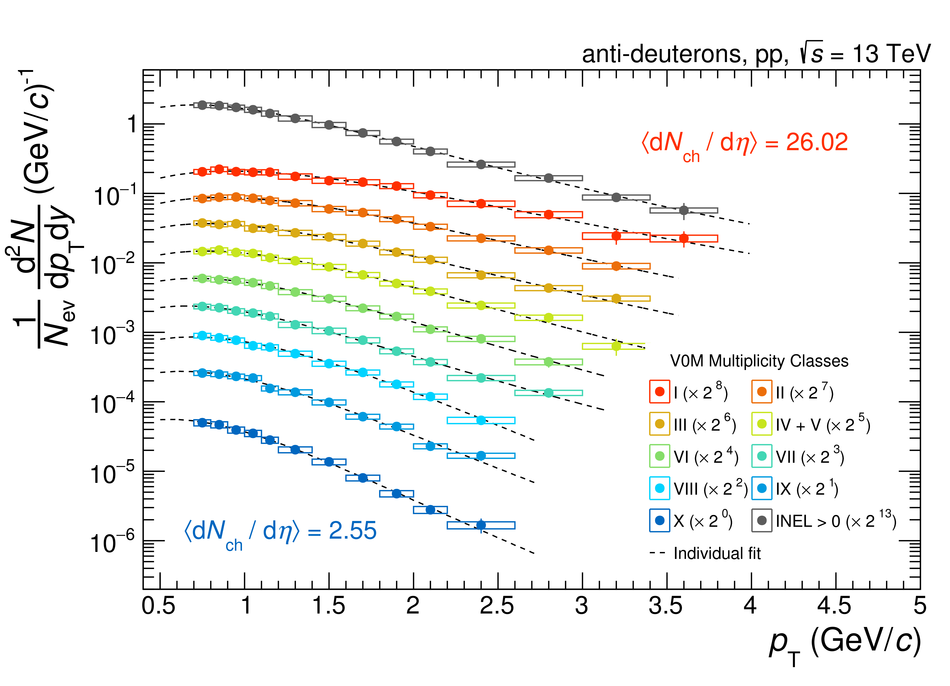# (Anti-)Deuteron production in pp collisions at $\sqrt{s}=13$ TeV

The study of (anti-)deuteron production in pp collisions has proven to be a powerful tool to investigate the formation mechanism of loosely bound states in high energy hadronic collisions. In this paper the production of $\text{(anti-)deuterons}$ is studied as a function of the charged particle multiplicity in inelastic pp collisions at $\sqrt{s}=13$ TeV using the ALICE experiment. Thanks to the large accumulated integrated luminosity, it has been possible to measure (anti-)deuteron production in pp collisions up to the same charged particle multiplicity ($\rm{d} N_{ch}/\rm{d}\eta\sim26$) as measured in p-Pb collisions at similar centre-of-mass energies. Within the uncertainties, the deuteron yield in pp collisions resembles the one in p-Pb interactions, suggesting a common formation mechanism behind the production of light nuclei in hadronic interactions. In this context the measurements are compared with the expectations of coalescence and Statistical Hadronisation Models (SHM).

Submitted to: EPJC
e-Print: arXiv:2003.03184 | PDF | inSPIRE
CERN-EP-2020-025

Figures

## Figure 1

 Transverse-momentum spectra of deuterons (top) and anti-deuterons (bottom) measured in pp collisions at $\sqrt{s}~=~$13~TeV in different multiplicity classes and in INEL$>$0 events. The mean charged-particle multiplicity for classes I and X are reported in the figures and all the values for the multiplicity classes can be found in Table \ref{tab:yields}. The statistical uncertainties are represented by vertical bars while the systematic uncertainties are represented by boxes. The dashed lines are individual fits with a L\'evy-Tsallis function~.## Figure 2

 Ratio between the transverse momentum spectra of anti-deuterons and deuterons in different multiplicity classes. The statistical uncertainties are represented by vertical bars while the systematic uncertainties are represented by boxes.## Figure 3

 Coalescence parameter $B_2$ for different multiplicity classes and for INEL$>$0 collisions. The statistical uncertainties are represented by vertical bars while the systematic uncertainties are represented by boxes. $B_2$ is shown as a function of $\pt/A$, being $A~=~2$ the mass number of the deuteron.## Figure 4

 c## Figure 5

 Ratio between the \pt-integrated yields of deuterons and protons for different multiplicities in pp collisions at $\sqrt{s}~=~13$~TeV and in $\sqrt{s}~=~7$~TeV~, in p--Pb collisions at $\snn~=~5.02$~TeV~ and in Pb--Pb collisions at $\snn~=~2.76$~TeV~. The statistical uncertainties are represented by vertical bars while the systematic uncertainties are represented by boxes. The two black lines are the theoretical predictions of the Thermal-FIST statistical model for two sizes of the correlation volume $V_C$, while the magenta line represents the expectation from a coalescence model